※ 半角英数字
年 ～
年

• 1

Initialising ...

Initialising ...

Initialising ...

Initialising ...

Initialising ...

Initialising ...

Initialising ...

Initialising ...

## The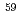Fe(n,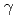)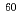Fe cross section from the surrogate ratio method and its effect on theFe nucleosynthesis

Yan, S. Q.*; Li, X. Y.*; 西尾 勝久; Lugaro, M.*; Li, Z. H.*; 牧井 宏之; Pignatari, M.*; Wang, Y. B.*; Orlandi, R.; 廣瀬 健太郎; et al.

Astrophysical Journal, 919(2), p.84_1 - 84_7, 2021/10

被引用回数：1 パーセンタイル：17.16(Astronomy & Astrophysics)

The long-livedFe (with a half-life of 2.62 Myr) is a crucial diagnostic of active nucleosynthesis in the Milky Way galaxy and in supernovae near the solar system. The neutron-capture reactionFe(n,)Fe onFe (half-life=44.5 days) is the key reaction for the production ofFe in massive stars. This reaction cross section has been previously constrained by the Coulomb dissociation experiment, which offered partial constraint on the E1-ray strength function but a negligible constraint on the M1 and E2 components. In this work, for the first time, we use the surrogate ratio method to experimentally determine theFe(n,)Fe cross sections in which all the components are included. We derived a Maxwellian-averaged cross section of 27.5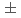3.5 mb at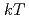= 30 keV and 13.41.7 mb at= 90 keV, roughly 10%-20% higher than previous estimates. We analyzed the impact of our new reaction rates in nucleosynthesis models of massive stars and found that uncertainties in the production ofFe from theFe(n,)Fe rate are at most 25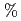. We conclude that stellar physics uncertainties now play a major role in the accurate evaluation of the stellar production ofFe.

## The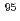Zr(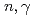)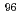Zr cross section from the surrogate ratio method and its effect on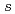-process nucleosynthesis

Yan, S. Q.*; Li, Z. H.*; Wang, Y. B.*; 西尾 勝久; Lugaro, M.*; Karakas, A. I.*; 牧井 宏之; Mohr, P.*; Su, J.*; Li, Y. J.*; et al.

Astrophysical Journal, 848(2), p.98_1 - 98_8, 2017/10

被引用回数：5 パーセンタイル：24.24(Astronomy & Astrophysics)

TheZr()Zr reaction cross section is crucial in the modelling of-process nucleosynthesis in asymptotic giant branch stars because it controls the operation of the branching point at the unstableZr and the subsequent production ofZr. We have carried out the measurement of the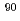Zr(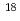O,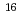O) and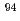Zr(O,O) reactions and obtained the-decay probability ratio of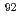Zr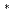andZrto determine theZr()Zr reaction cross sections with the surrogate ratio method. We tested our deduced maxwellian-averaged cross section in stellar models with masses between 2 and 6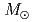and metallicities 0.014 and 0.03. The largest changes - up 80 % variations inZr - are seen in the models of 3-4, where the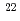Ne neutron source is mildly activated. The new rate can still provide a match to data from meteoritic stardust silicon carbide grains, provided the maximum mass of the parent stars is below 4, for a metallicity of 0.03.

## Examination of the surrogate ratio method for the determination of the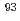Zr(n,)Zr cross section with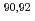Zr(O,O)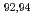Zr reactions

Yan, S. Q.*; Li, Z. H.*; Wang, Y. B.*; 西尾 勝久; 牧井 宏之; Su, J.*; Li, Y. J.*; 西中 一朗; 廣瀬 健太郎; Han, Y. L.*; et al.

Physical Review C, 94(1), p.015804_1 - 015804_5, 2016/07

被引用回数：6 パーセンタイル：47.4(Physics, Nuclear)

The relative-decay probability ratios of the neutron resonance states inZr andZr populated via two neutron transfer reactions,Zr(O,O)Zr andZr(O,O)Zr, have been measured to test the validity of the surrogate ratio method (SRM) in determining the (n,) reaction cross section. The cross sections of theZr(n,)Zr reaction are derived from the experimentally obtained ratios and the cross sections of the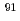Zr(n,)Zr reaction in the equivalent neutron energy range of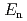= 0 - 8 MeV. The deduced cross sections ofZr(n,)Zr reaction agree with the directly measured ones in the low-energy region, and with the evaluated ENDF/B-VII.1 data at higher energies of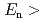3 MeV. The agreement supports the concept of the SRM method to indirectly determine the (n,) reaction cross sections.

3 件中 1件目～3件目を表示
• 1